Area bounded by curve and horizontal or vertical line

Chapter 8 Class 12 Application of Integrals
Concept wise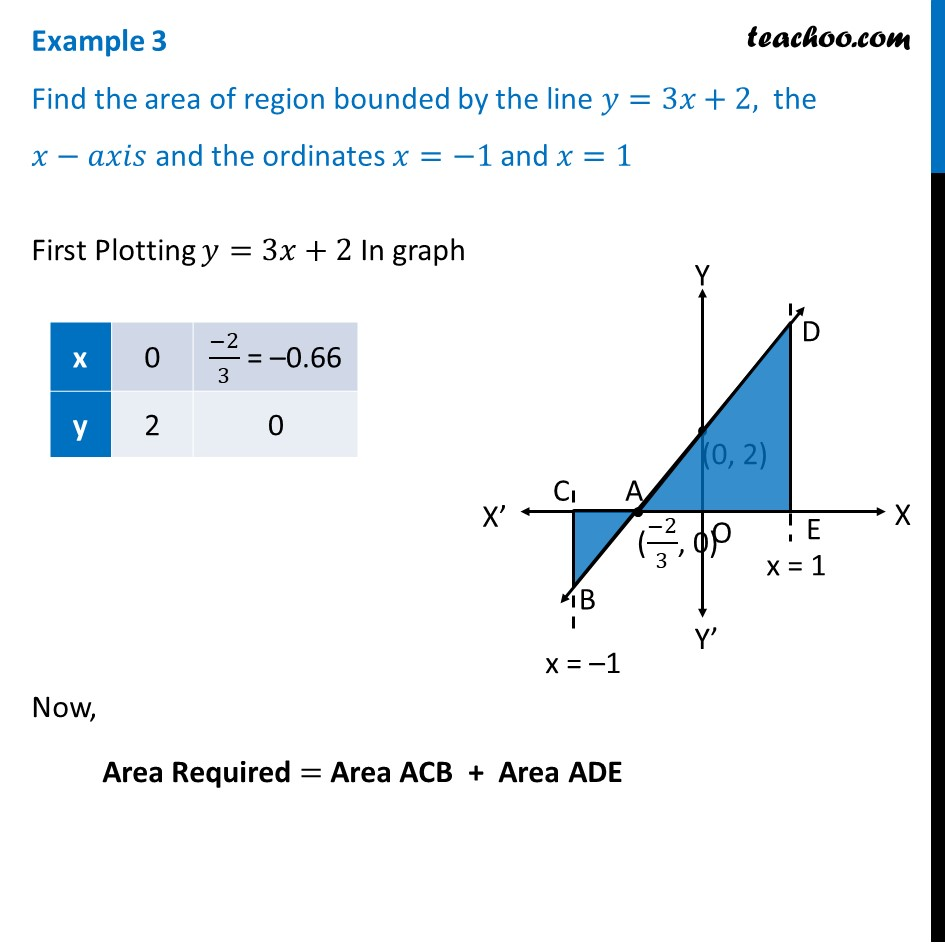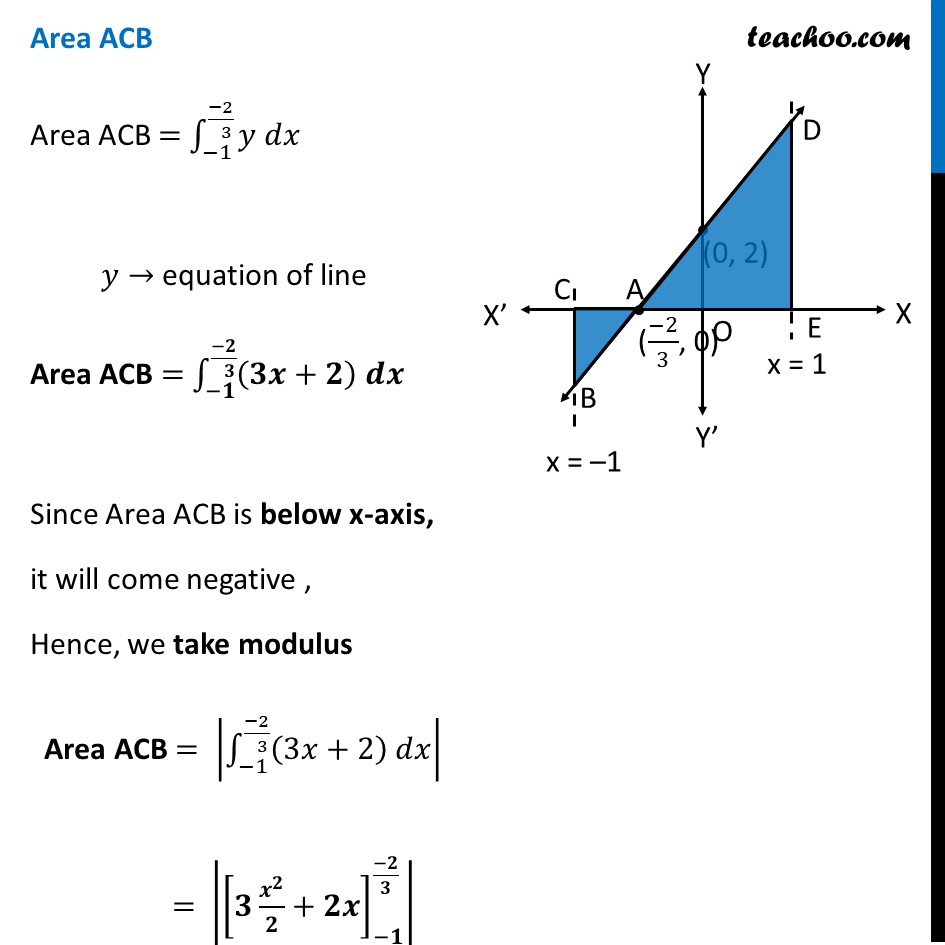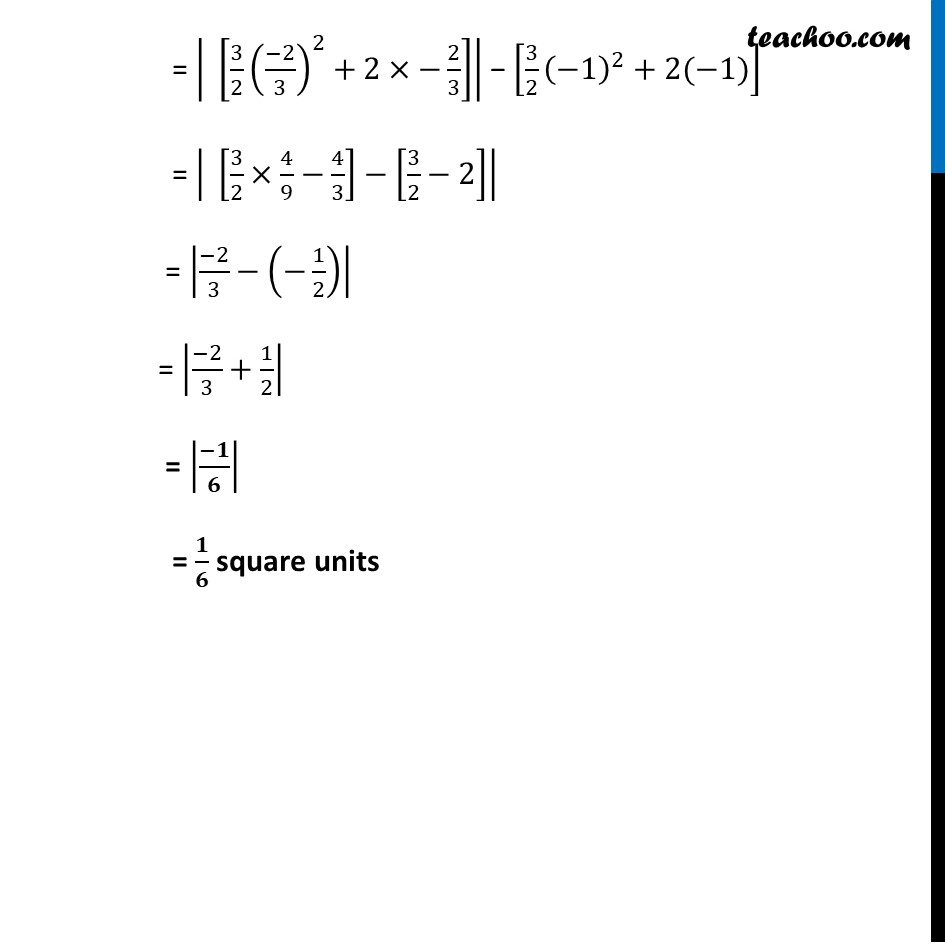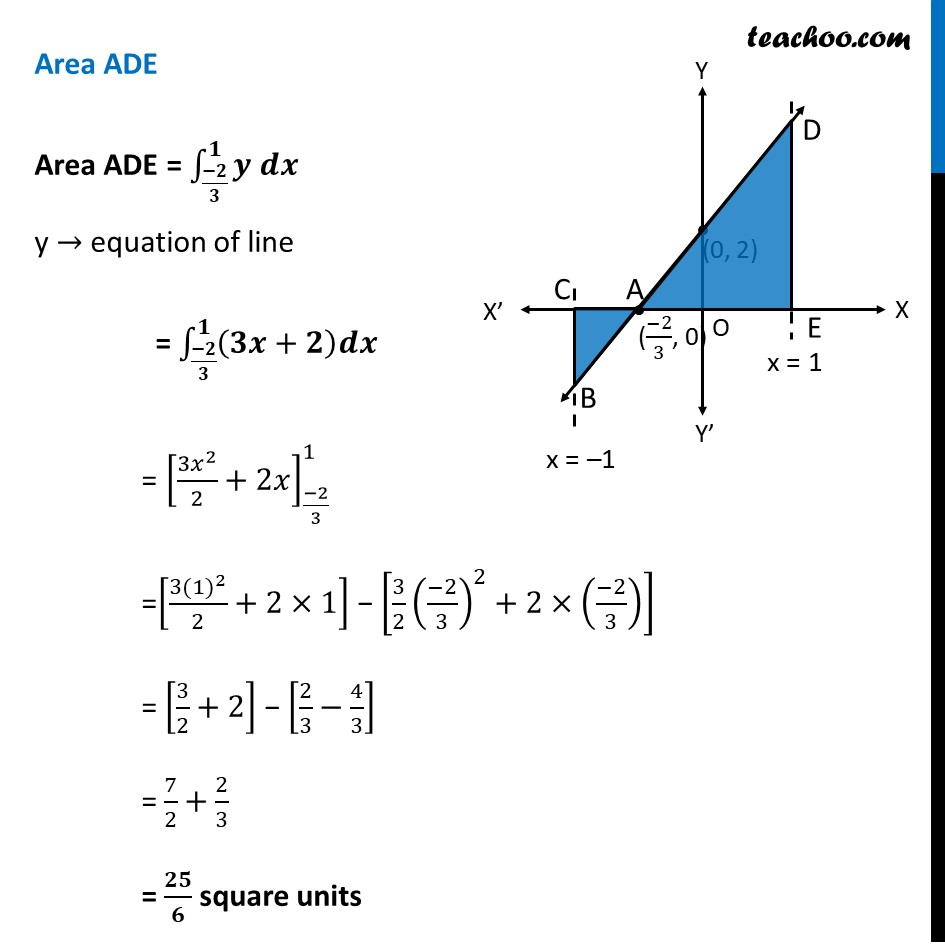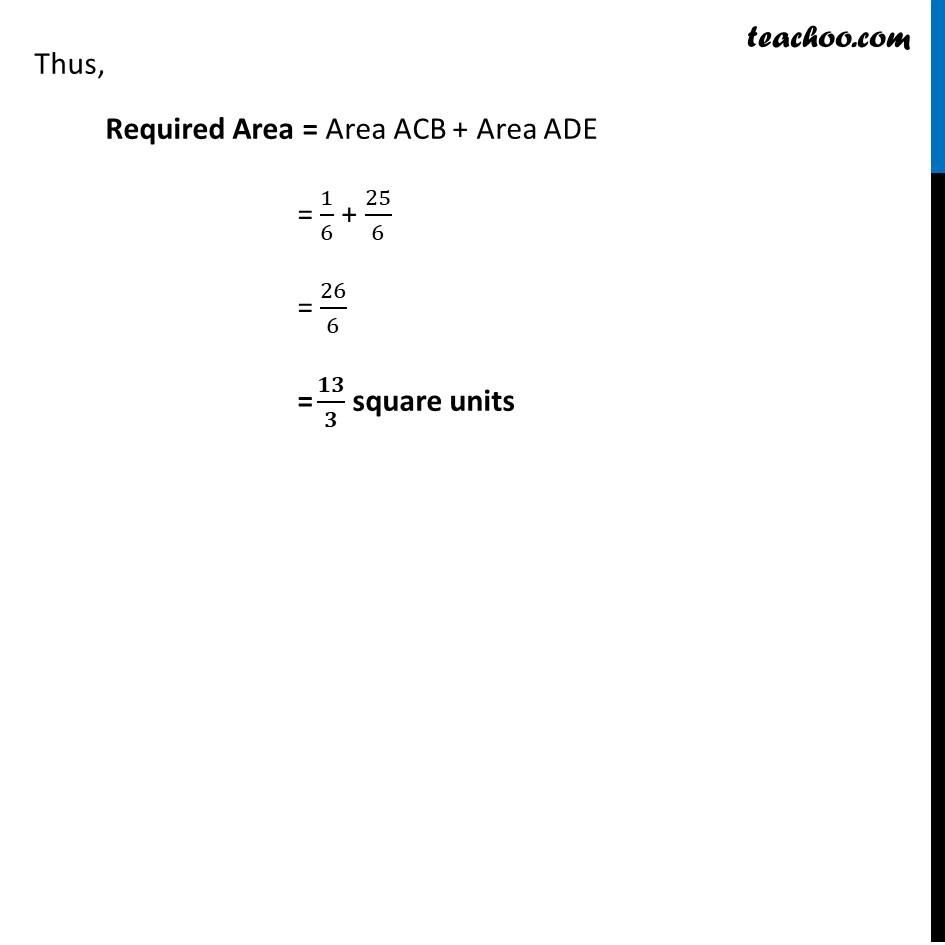Learn in your speed, with individual attention - Teachoo Maths 1-on-1 Class

### Transcript

Example 3 Find the area of region bounded by the line 𝑦=3𝑥+2, the 𝑥−𝑎𝑥𝑖𝑠 and the ordinates 𝑥=−1 and 𝑥=1 First Plotting 𝑦=3𝑥+2 In graph Now, Area Required = Area ACB + Area ADE Area ACB Area ACB = ∫_(−1)^((−2)/( 3))▒〖𝑦 𝑑𝑥〗 𝑦→ equation of line Area ACB = ∫_(−𝟏)^((−𝟐)/( 𝟑))▒〖(𝟑𝒙+𝟐) 𝒅𝒙〗 Since Area ACB is below x-axis, it will come negative , Hence, we take modulus Area ACB = |∫_(−1)^((−2)/( 3))▒〖(3𝑥+2) 𝑑𝑥〗| = |[𝟑 𝒙^𝟐/𝟐+𝟐𝒙]_(−𝟏)^((−𝟐)/𝟑) | = |" " [3/2 ((−2)/3)^2+2×−2/3]| − [3/2 (−1)^2+2(−1)] = |" " [3/2×4/9−4/3]−[3/2−2]| = |(−2)/3−(−1/2)| = |(−2)/3+1/2| = |(−𝟏)/𝟔| = 𝟏/𝟔 square units Area ADE Area ADE = ∫1_((−𝟐)/𝟑)^𝟏▒〖𝒚 𝒅𝒙〗 y → equation of line = ∫1_((−𝟐)/𝟑)^𝟏▒(𝟑𝒙+𝟐)𝒅𝒙 = [(3𝑥^2)/2+2𝑥]_((−2)/3)^1 =[(3〖(1)〗^2)/2+2×1] − [3/2 ((−2)/3)^2+2×((−2)/3)] = [3/2+2] − [2/3−4/3] = 7/2+2/3 = 𝟐𝟓/𝟔 square units Thus, Required Area = Area ACB + Area ADE = 1/6 + 25/6 = 26/6 = 𝟏𝟑/𝟑 square units